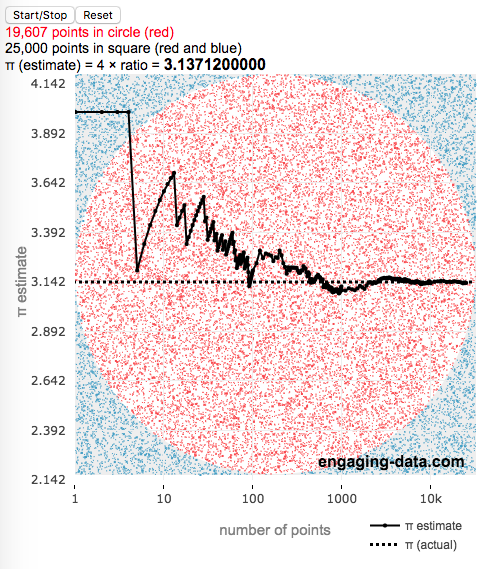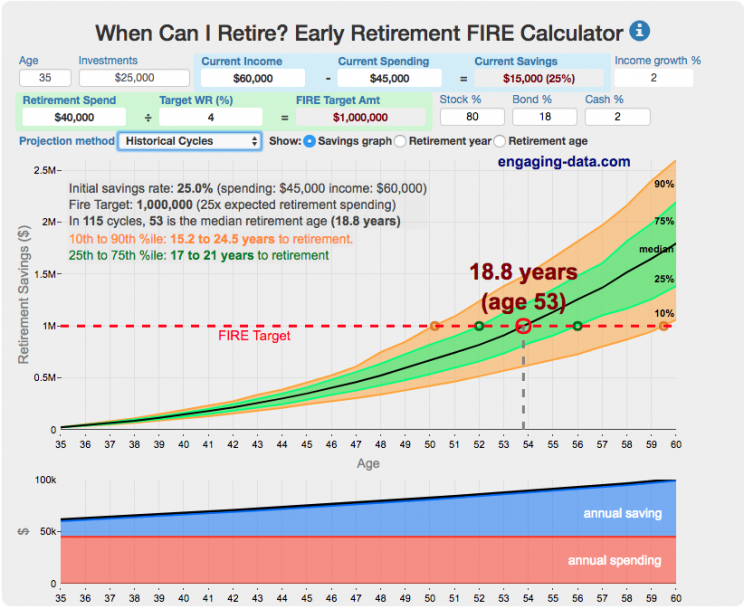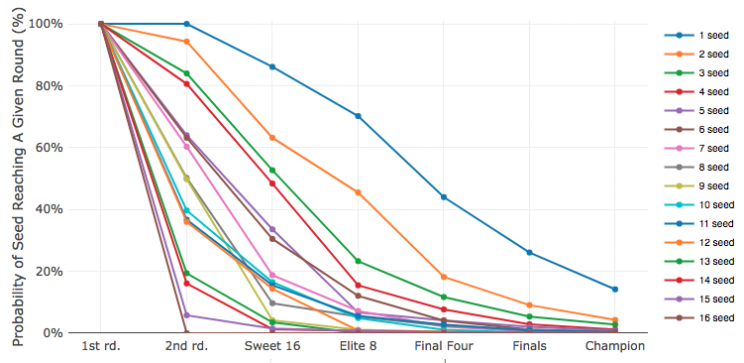# Posts for Tag: Monte Carlo simulation

### Estimating pi (π) using Monte Carlo Simulation

Posted In: MathThis interactive simulation estimates the value of the fundamental constant, pi (π), by drawing lots of random points to estimate the relative areas of a square and an inscribed circle.

Pi, (π), is used in a number of math equations related to circles, including calculating the area, circumference, etc. and is widely used in geometry, trigonometry and physics.

This app estimates the value of pi by comparing the area of a square and an inscribed circle. The areas are calculated by randomly placing dots into the square and then counting how many of them are also inside the circle. If you do this enough times, you will get a rough ratio of the relative areas of the two shapes. These points are plotted on the graph (red if the fall inside the circle and blue if the fall outside).

Also shown on the graph is the value of our estimate of pi as the simulation progresses, from a few points to many thousands, to millions of points. We can see that when we have only a few points, the value may not be very accurate but as the number of points increases the value of our estimate gets closer to the true value. Running the simulation will add and plot 1 million points. After the first 100 points are added, the rate at which points are added increases. You’ll notice this as the speed at which dots fills up the square increases and because the plot is shown with a logarithmic x-axis.

Here is the math:
Length of side of square: $2 \times r$
radius of circle: $r$
Area of square: $A_{square} = 4r^2$
Area of circle: $A_{circle} = \pi r^2$

The ratio of areas is $A_{circle}/A_{square} = \pi r^2 / 4r^2 = \pi / 4$
Solving for pi: $\pi = 4 \times A_{circle}/A_{square} \approx 4 \times N_{dots_{circle}}/N_{dots_{square}}$
So pi is estimated as 4 times the ratio of dots in the circle vs square

Tools:
This was programmed in javascript, canvas and plotted using the open source plotly javascript plotting library.### When Can I Retire? Early Retirement Calculator / FIRE Calculator

Posted In: Financial Independence | Money#### How long do I need to save before I can retire?This early retirement calculator / visualizer is designed to project the number of years until you can retire, based upon a few key inputs such as annual income and spending, income growth rate, expected annual spending in retirement and asset allocation. It is a pre-retirement calculator that is useful before you retire to get a sense of how many years it is likely to take to accumulate enough money to retire. The three primary modes that are available in the early retirement calculator are: (1) constant, single fixed-percentage real return rates, (2) historical series of real returns are applied to account for likely variability in future returns and (3) monte carlo simulation of the variable returns based upon user-specified input parameters.

This interactive calculator was built to let you play with the inputs and help you understand how savings rate and retirement spending strongly determine how long it will take you to save up for retirement. Note: it does not simulate the post-retirement period when you start to draw down your savings. That can be done on this post-retirement calculator (Rich, Broke or Dead) which compares the frequency of various outcomes in retirement (running out of money, ending up with way too much money, and life-expectancy).
(more…)

### March Madness Bracket Picker Based on Historical Probabilities (and Monte Carlo Simulation)

Posted In: Sports(more…)